# High School Physics : Electric Circuits

## Example Questions

### Example Question #11 : Use Ohm's Law

Ohm's Law describes the relationship between which variables?

Voltage, current, resistance

Resistance, power, electromotive force

Voltage, electric field, power

Electric field, current, power

Power, resistivity, electromotive force

Voltage, current, resistance

Explanation:

Ohm's law can be written as: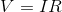It demonstrates that voltage is equal to the product of current and resistance within an electric circuit.

### Example Question #11 : Circuit Calculations And Concepts

The current in a circuit is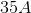and the voltage is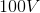. What is the resistance?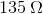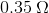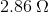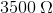Explanation:

Ohm's law says. Plug in our given values to solve.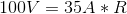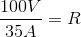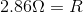### Example Question #11 : Electric Circuits

What is the current of a circuit with a voltage of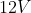and a total resistance of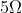?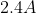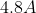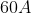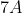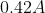Explanation:

For this problem, use Ohm's law: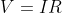. In this equation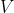is the voltage,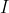is the current, and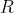is the resistance.

We can re-arrange the equation to solve specifically for.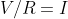Plug in the given values for voltage and resistance to solve for the current.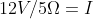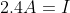### Example Question #12 : Electric Circuits

What is the resistance in a circuit with a voltage of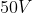and a current of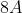?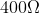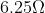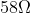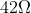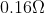Explanation:

For this problem, use Ohm's law:. In this equationis the voltage,is the current, andis the resistance.

We can re-arrange the equation to solve specifically for.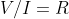Plug in the given values for voltage and current and solve for resistance.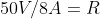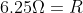### Example Question #11 : Electricity And Magnetism

What is the voltage of a circuit with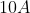of current and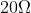of resistance?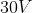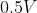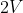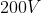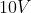Explanation:

For this problem, use Ohm's law:. In this equationis the voltage,is the current, andis the resistance.

Plug in the given values and solve for the voltage.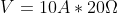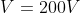### Example Question #11 : Circuit Calculations And Concepts

Ohm's Law describes the relationship between which variables?

Power, resistivity, electromotive force

Electric field, current, power

Resistance, power, electromotive force

Voltage, current, resistance

Voltage, electric field, power

Voltage, current, resistance

Explanation:

Ohm's law can be written as:It demonstrates that voltage is equal to the product of current and resistance within an electric circuit.

### Example Question #665 : High School Physics

A circuit has a current,, a voltage,, and a resistance,. If the voltage remains constant, but the current is doubled (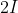), what must the new resistance be?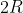There is insufficient information to solve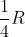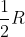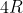Explanation:

To solve this problem, use Ohm's law:Since we are doubling the current, but the voltage is remaining the same, we can set our old and new equations equal to each other.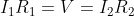We know that the second current is equal to twice the first current.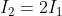Use this equation to substitute current into the first equation.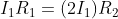The initial current now cancels out from both sides.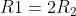Divide both sides by two to isolate the final resistance variable.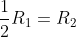### Example Question #11 : Electricity And Magnetism

What is the power of a circuit with a current of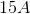and a resistance of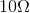?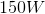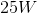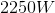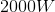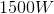Explanation:

The standard equation for power is: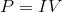We are given only current and reistance, meaning we must manipulate this equation so that only those variables are present. To do this, we can substitute Ohm's law for voltage.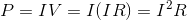Now we have an equation for power that uses only current and resistance. Plug in the values given in the question to find the final answer.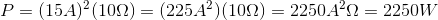### Example Question #11 : Electricity And Magnetism

Each branch of a parallel circuit carries an equal __________.

resistance

power

current

voltage

capacitance

voltage

Explanation:

By definition, each branch of a parallel circuit will have the same voltage. The current, however, may be split between branches in inequal amounts. In contrast, a series circuit will carry a constant current throughout the circuit, but voltage may differ between elements.

Resistance and capacitance are dependent on the elements present in a given circuit, so no claims can be made. Power is similarly dependent on the elements present in a specific circuit.

### Example Question #1 : Understanding Series And Parallel

Each component of a series circuit carries an equal __________.

capacitance

voltage

current

power

resistance